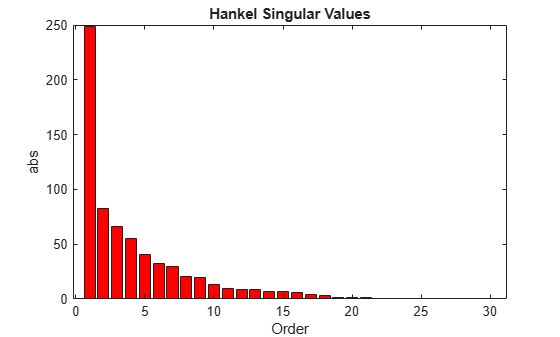Documentation

## Hankel Singular Values

In control theory, eigenvalues define a system stability, whereas Hankel singular values define the “energy” of each state in the system. Keeping larger energy states of a system preserves most of its characteristics in terms of stability, frequency, and time responses. Model reduction techniques presented here are all based on the Hankel singular values of a system. They can achieve a reduced-order model that preserves the majority of the system characteristics.

Mathematically, given a stable state-space system (A,B,C,D), its Hankel singular values are defined as 

`${\sigma }_{H}=\sqrt{{\lambda }_{i}\left(PQ\right)}$`

where P and Q are controllability and observability grammians satisfying

`$\begin{array}{l}AP+P{A}^{T}=-B{B}^{T}\\ {A}^{T}Q+QA=-{C}^{T}C.\end{array}$`

For example, generate a random 30-state system and plot its Hankel singular values.

```rng(1234,'twister'); G = rss(30,4,3); hankelsv(G)```The plot shows shows that system `G` has most of its “energy” stored in states 1 through 15 or so. Later, you will see how to use model reduction routines to keep a 15-state reduced model that preserves most of its dynamic response.

## Related Examples